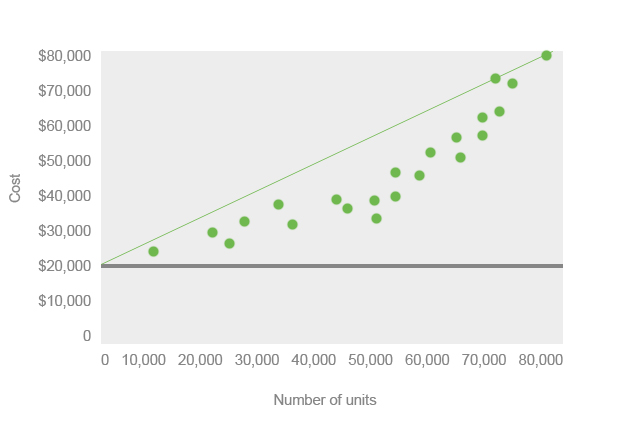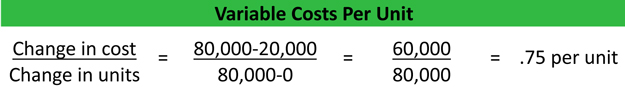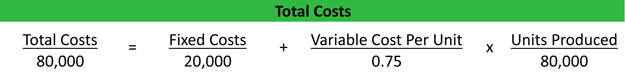# What is the High-Low Method?

Definition: The high-low method is a technique managerial accountants use to estimate the mixed production costs at various levels of production by calculating the variable cost rate and total fixed costs. In other words, it’s a formula used by management to split the fixed and variable costs associated with producing a good and chart out these data points. A line is then drawn connecting the lowest and highest points to represent the average.

## What Does High Low Method Mean?

What is the definition of the high-low method? This method can also be used to chart out all the purchases of goods and their prices. A straight line on the graph connects the highest price and lowest price of goods. Look at this example. The lowest price is the fixed price of \$20,000. The highest price is \$80,000.The variable cost per unit can be found using a simple slope formula. Change in y divided by the change in x. According to the formula below, the variable cost per unit is 75 cents.Now management can figure out how much it will cost to produce any amount of products. All they have to do is look at the fixed costs plus the variable cost per unit multiplied by the units produced. Rearranging this formula, management can also figure out what total fixed costs are if they were unknown.The high low method is very useful for helping management determine not only what total costs will be, but also how much of a certain product to produce. There are a few shortfalls to the high low method. For instance, it does not recognize any other costs except the highest and lowest costs.

Keep in mind that this method is far less precise than other cost methods like the least-squares method. It’s a simply and easy way to understand the relationship between fixed and variable costs at different levels of output.

## Summary Definition

Define High Low Method: High-low method means a graphical approach to determining the average cost of purchasing a good or producing a product by plotting all transactions and connecting the lowest and highest points.